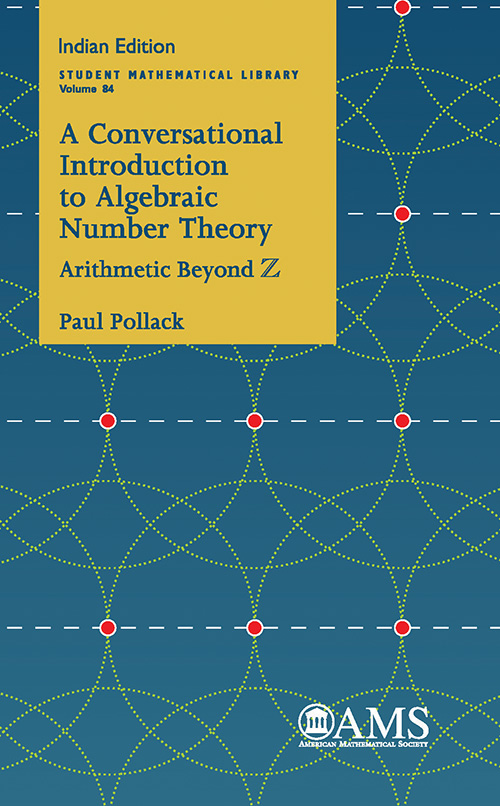## A Conversational Introduction to Algebraic Number Theory

#### About the Book

Gauss famously referred to mathematics as the “queen of the sciences” and to number theory as the “queen of mathematics”. This book is an introduction to algebraic number theory, meaning the study of arithmetic in finite extensions of the rational number field Q. Originating in the work of Gauss, the foundations of modern algebraic number theory are due to Dirichlet, Dedekind, Kronecker, Kummer, and others. This book lays out basic results, including the three “fundamental theorems”: unique factorization of ideals, finiteness of the class number, and Dirichlet’s unit theorem. While these theorems are by now quite classical, both the text and the exercises allude frequently to more recent developments.

In addition to traversing the main highways, the book reveals some remarkable vistas by exploring scenic side roads. Several topics appear that are not present in the usual introductory texts. One example is the inclusion of an extensive discussion of the theory of elasticity, which provides a precise way of measuring the failure of unique factorization.

The book is based on the author’s notes from a course delivered at the University of Georgia; pains have been taken to preserve the conversational style of the original lectures.

#### Contributors (Author(s), Editor(s), Translator(s), Illustrator(s) etc.)

Paul Pollack: University of Georgia, Athens, GA

• Preface 8
• Chapter 1. Getting our feet wet 12
• Chapter 2. Cast of characters 20
• Chapter 3. Quadratic number fields: First steps 30
• Chapter 4. Paradise lost —and found 38
• Chapter 5. Euclidean quadratic fields 48
• Chapter 6. Ideal theory for quadratic fields 60
• Chapter 7. Prime ideals in quadratic number rings 70
• Chapter 8. Units in quadratic number rings 78
• Chapter 9. A touch of class 90
• Chapter 10. Measuring the failure of unique factorization 102
• Chapter 11. Euler's prime-producing polynomial and the criterion of Frobenius–Rabinowitsch 116
• Chapter 12. Interlude: Lattice points 128
• Chapter 13. Back to basics: Starting over with arbitrary number fields 140
• Chapter 14. Integral bases: From theory to practice, and back 154
• Chapter 15. Ideal theory in general number rings 170
• Chapter 16. Finiteness of the class group and the arithmetic of \Z 182
• Chapter 17. Prime decomposition in general number rings 190
• Chapter 18. Dirichlet;s unit theorem, I 206
• Chapter 19. A case study: Units in \Z[v2] and the Diophantine equation X3-2Y3±1       216
• Chapter 20. Dirichlet's unit theorem, II 226
• Chapter 21. More Minkowski magic, with a cameo appearance by Hermite 236
• Chapter 22. Dedekind's discriminant theorem 252
• Chapter 23. The quadratic Gauss sum 266
• Chapter 24. Ideal density in quadratic number fields 282
• Chapter 25. Dirichlet's class number formula 292
• Chapter 26. Three miraculous appearances of quadratic class numbers 306
• Index 324

`